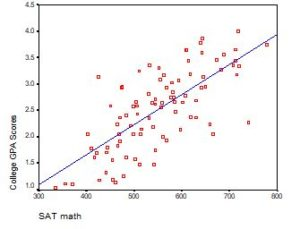# Interpreting intercept

### Interpreting the Intercept in a Regression Model

February 21st, 2023 by

Interpreting the Intercept in a regression model isn’t always as straightforward as it looks.

Here’s the definition: the intercept (often labeled the constant) is the expected value of Y when all X=0. But that definition isn’t always helpful. So what does it really mean?

### Regression with One Predictor XIf X sometimes equals 0, the intercept is simply the expected value of Y at that value. In other words, it’s the mean of Y at one value of X. That’s meaningful.

If X never equals 0, then the intercept has no intrinsic meaning. You literally can’t interpret it. That’s actually fine, though. You still need that intercept to give you unbiased estimates of the slope and to calculate accurate predicted values. So while the intercept has a purpose, it’s not meaningful.

Both these scenarios are common in real data. (more…)

### The Impact of Removing the Constant from a Regression Model: The Categorical Case

December 9th, 2016 byIn a simple linear regression model, how the constant (a.k.a., intercept) is interpreted depends upon the type of predictor (independent) variable.

If the predictor is categorical and dummy-coded, the constant is the mean value of the outcome variable for the reference category only. If the predictor variable is continuous, the constant equals the predicted value of the outcome variable when the predictor variable equals zero.

### Removing the Constant When the Predictor Is Categorical

When your predictor variable X is categorical, the results are logical. Let’s look at an example. (more…)

### Interpreting (Even Tricky) Regression Coefficients – A Quiz

January 15th, 2010 by

Here’s a little quiz:

### True or False?

1. When you add an interaction to a regression model, you can still evaluate the main effects of the terms that make up the interaction, just like in ANOVA.

2. The intercept is usually meaningless in a regression model. (more…)